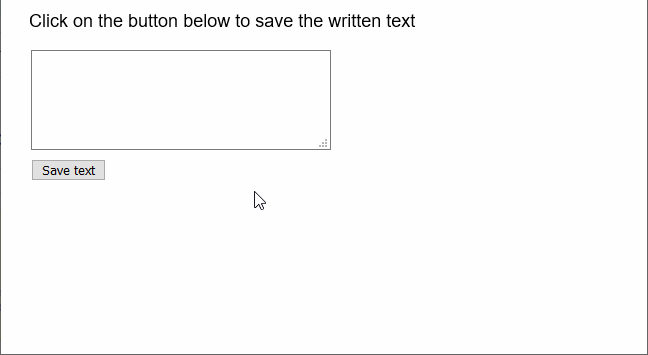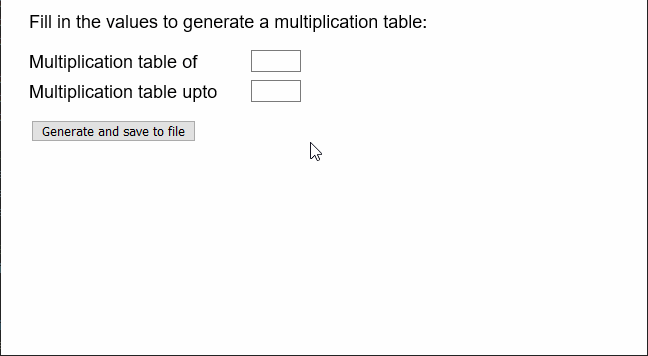# p5.js createWriter() Function

The createWriter() function in p5.js is used to create a p5.PrintWriter object that can be used to write or print to various available streams.

Syntax:

`createWriter( name, [extension] )`

Parameters: This function accepts two parameters as mentioned above and described below.

• name: It is a string that denotes the name of the file to be created.
• extension: It is a string that specifies the extension of the file. It is an optional parameter.

Return Value: It returns a p5.PrintWriter object that denotes the writer.

The example below illustrates the createWriter() function in p5.js:

Example 1:

 `let fwriter; ` ` `  `function` `setup() { ` `  ``createCanvas(600, 300); ` `  ``textSize(18); ` ` `  `  ``// Create a textarea for the input of text ` `  ``inputArea = createElement(``"textarea"``); ` `  ``inputArea.position(30, 50); ` `  ``inputArea.size(300, 100); ` ` `  `  ``// Create a button for saving text ` `  ``saveBtn = createButton(``"Save text"``); ` `  ``saveBtn.position(30, 160); ` `  ``saveBtn.mousePressed(saveFile); ` ` `  `  ``// Setup the writer ` `  ``fwriter = createWriter(``"note.txt"``); ` ` `  `  ``text(``"Click on the button below to save the written text"``, 20, 20); ` `} ` ` `  `function` `saveFile() { ` `  ``// Get the value of the textarea ` `  ``// and split the strings on the basis ` `  ``// of the nextline character ` `  ``stringList = inputArea.value().split(``"\n"``); ` ` `  `  ``// Save the strings to file ` `  ``for` `(line of stringList) { ` `    ``fwriter.print(line); ` `  ``} ` ` `  `  ``// Close the writer ` `  ``fwriter.close(); ` ` `  `  ``// Clear the writer ` `  ``fwriter.clear(); ` `} `

Output:Example 2:

 `function` `setup() { ` `  ``createCanvas(600, 300); ` `  ``textSize(18); ` ` `  `  ``// Create two inputs for the ` `  ``// multiplication table ` `  ``multiOf = createInput(); ` `  ``multiOf.position(250, 50); ` `  ``multiOf.size(50); ` ` `  `  ``multiTo = createInput(); ` `  ``multiTo.position(250, 80); ` `  ``multiTo.size(50); ` ` `  `  ``// Create a button for saving text ` `  ``saveBtn = createButton(``"Generate and save to file"``); ` `  ``saveBtn.position(30, 120); ` `  ``saveBtn.mousePressed(saveFile); ` ` `  `  ``// Setup the writer ` `  ``fwriter = createWriter(``"tables.txt"``); ` `} ` ` `  `function` `draw() { ` `  ``clear(); ` `  ``text(``"Fill in the values to generate a multiplication table:"``, 20, 20); ` `  ``text(``"Multiplication table of"``, 20, 60); ` `  ``text(``"Multiplication table upto"``, 20, 90); ` `} ` ` `  `function` `saveFile() { ` `  ``// Get the value of the two inputs ` `  ``// and generate table ` `  ``let multipicand = multiOf.value(); ` `  ``let multiMax = multiTo.value(); ` ` `  `  ``for` `(let multiplier = 1; multiplier <= multiMax; multiplier++) { ` `    ``let textToWrite = ` `      ``multipicand + ``" * "` `+ multiplier + ``" = "` `+ multipicand * multiplier; ` ` `  `    ``// Print to the writer ` `    ``fwriter.print(textToWrite); ` `  ``} ` ` `  `  ``// Close the writer ` `  ``fwriter.close(); ` ` `  `  ``// Clear the writer ` `  ``fwriter.clear(); ` `} `

Output:Online editor: https://editor.p5js.org/

Whether you're preparing for your first job interview or aiming to upskill in this ever-evolving tech landscape, GeeksforGeeks Courses are your key to success. We provide top-quality content at affordable prices, all geared towards accelerating your growth in a time-bound manner. Join the millions we've already empowered, and we're here to do the same for you. Don't miss out - check it out now!